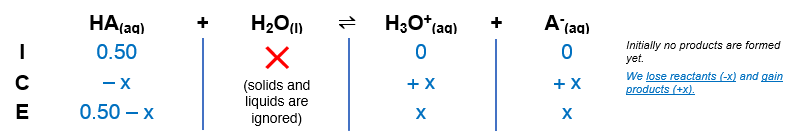# Problem: A 0.50 M solution of an acid HA has pH = 2.24. What is the value of Ka for the acid?

###### FREE Expert Solution

The dissociation of HNO2 is as follows:

HA(aq) + H2O(l)  H3O+(aq) + A(aq)

From this, we can construct an ICE table. Remember that liquids are ignored in the ICE table and Ka expression.The Ka expression for HA is:

$\overline{){{\mathbf{K}}}_{{\mathbf{a}}}{\mathbf{=}}\frac{\mathbf{products}}{\mathbf{reactants}}{\mathbf{=}}\frac{\mathbf{\left[}{\mathbf{H}}_{\mathbf{3}}{\mathbf{O}}^{\mathbf{+}}\mathbf{\right]}\mathbf{\left[}{\mathbf{A}}^{\mathbf{-}}\mathbf{\right]}}{\mathbf{\left[}\mathbf{HA}\mathbf{\right]}}}$

94% (42 ratings)###### Problem Details

A 0.50 M solution of an acid HA has pH = 2.24. What is the value of Ka for the acid?

Frequently Asked Questions

What scientific concept do you need to know in order to solve this problem?

Our tutors have indicated that to solve this problem you will need to apply the Weak Acids concept. If you need more Weak Acids practice, you can also practice Weak Acids practice problems.

What professor is this problem relevant for?

Based on our data, we think this problem is relevant for Professor Islam's class at SJU.